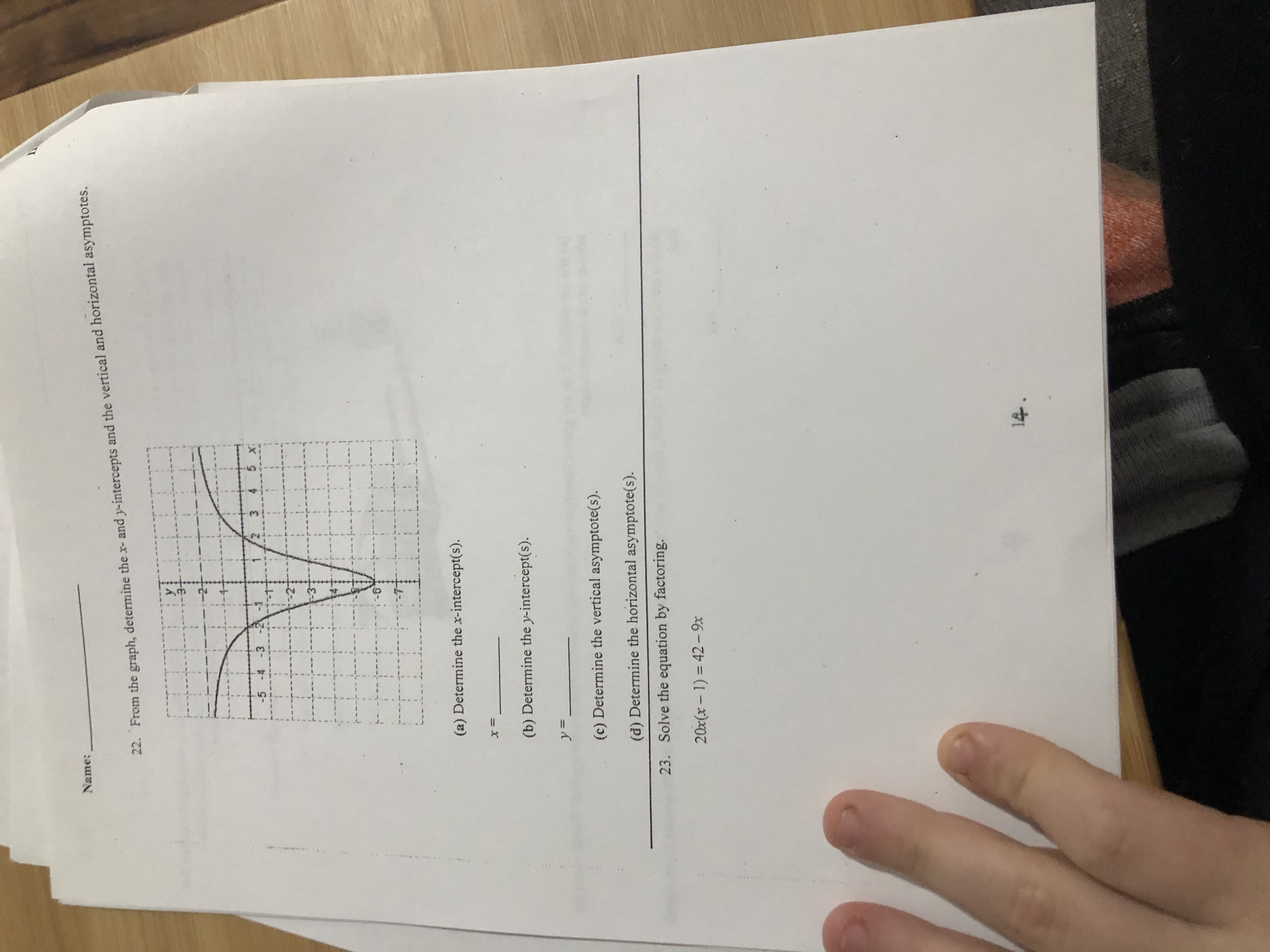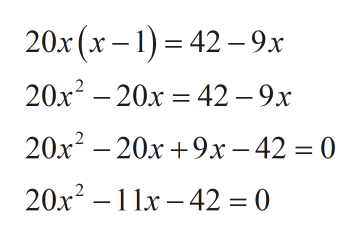# Name:22. From the graph, determine the x- and y-intercepts and the vertical and horizontal asymptotes.y:-23 4 5 x-5 -4 -3(a) Determine the x-intercept(s).(b) Determine the y-intercept(s).y= _(c) Determine the vertical asymptote(s).(d) Determine the horizontal asymptote(s).Solve the equation by factoring.23.20x(x - 1) = 42- 9x%3D14.

Question
1 viewshelp_outlineImage TranscriptioncloseName: 22. From the graph, determine the x- and y-intercepts and the vertical and horizontal asymptotes. y: -2 3 4 5 x -5 -4 -3 (a) Determine the x-intercept(s). (b) Determine the y-intercept(s). y= _ (c) Determine the vertical asymptote(s). (d) Determine the horizontal asymptote(s). Solve the equation by factoring. 23. 20x(x - 1) = 42- 9x %3D 14. fullscreen
check_circle

Step 1

Simplify the given expression to ...help_outlineImage Transcriptionclose20x(x – 1) = 42 – 9x 20x2 – 20x = 42 – 9x 20x? – 20x +9x- 42 = 0 20x? – 11x – 42 = 0 fullscreen

### Want to see the full answer?

See Solution

#### Want to see this answer and more?

Solutions are written by subject experts who are available 24/7. Questions are typically answered within 1 hour.*

See Solution
*Response times may vary by subject and question.
Tagged in

### Other